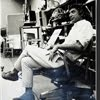## Eugene Ray SDSU 1970'sEugene Ray 1970's. photo credit: Tom Davis

## Monday, July 21, 2014

### INSIDE THE "SHIP'S" STUDIO / vital media invention timing

+MY LIFE'S WORK (OEUVRE)+
>is kept in dozens of ring binders that<
>i occasionally extract documenta to<                                                                                         >photograph for our nightly blog<
^^^^^^^^^^^^^^^^^^^^^^
+TONIGHT'S PHOTOS+
>were extracted from one binder today<
>and tonight's blog and a few nights<
+(FLASH OF LIGHT+
+/ EVENING SKY)+
>ahead is composed of these photos<
^^^^^^^^^^^^^^^^^^^^^^
+THIS EFFORT WAS GALVANIZED+
+BY YESTERDAY'S BLOG ESSAY+
>"silver ship lives/(current & early")<
+FOCUSED ON THE SILVER SHIP+
^^^^^^^^^^^^^^^^^^^^^^
>the architectural documenta i prefer are<
>the presentation drawings which in this<
+CALIFORNIA VILLAS)+
>case is a color xerographic collage<
^^^^^^^^^^^^^^^^^^^^^
>carol olten wrote this very generous<
>article on my work about the time<
+("S.D. UNION" / 1985+
+/ (CAROL OLTEN)+
>my work was exhibited in paris<
^^^^^^^^^^^^^^^^^^^^
>the documenta i rarely show because it<
>is so personal is newspaper interviews<
+(FIRST"SHIP"ARTICLE+
+/ ANDREA HOFFMAN)+
>even for the most part, very kind<
^^^^^^^^^^^^^^^^^^^^^
>for tulane dean john w. lawrence<
>who believed in me very early<
+(TULANE ESSENCE)+
>(as did bob heck at l.s.u.)<
^^^^^^^^^^^^^^^^^^^^
>my california villa designs were an<
>inspiration via plantation memory<
+PLANTS ESSENCE+
>(a reflection of genetic memory)<
^^^^^^^^^^^^^^^^^^^^^^^
>the tulane alumni magazine has twice<
>honored my work w/ kind articles<
+FOUCHER PLANTATION)+
>this is the second & most complete<
^^^^^^^^^^^^^^^^^^^^^^^^
>the first tulanean alumni magazine article<
>on my work surprised me as they found<
+("LAJOLLA LIGHT" NEWS+
+PAPER PHOTOS (REF.)+
>out about me via the lajolla light paper<
^^^^^^^^^^^^^^^^^^^^^^^^^^
>this los angeles times news article by<
>dirk sutro was well done and much<
+(LOS ANGELES TIMES /+
+DIRK SUTRO ARTICLE)+
>appreciated. he came to our campus<
^^^^^^^^^^^^^^^^^^^^^^
>photo collage xerography was the new<
>affordable color medium that allowed<
+(COLOR XEROGRAPHY+
+WAS OUR BLESSING)+
>me to document silver ship oeuvre<
^^^^^^^^^^^^^^^^^^^^^
>"sci arc" was a very good architectural school<
>when i lectured there and the director once<
+("S. CALIFORNIA INST+
+OF ARCHITECTURE")+
>took a helicopter to our sdsu grad seminar<
^^^^^^^^^^^^^^^^^^^^^^^^^^^
>you are invited here into the silver ship<
>studio to peer over my shoulder at the<
+(SILVER SHIP DESIGNS /+
^^^^^^^^^^^^^^^^^^^^^^^
>the frank lloyd wright school, taliesin<
>west, was one of the final venues for,
+(COLOR SLIDES WERE+
+MY LECTURE MEDIA)+
>my color slide lecture,(spring 1993)<
^^^^^^^^^^^^^^^^^^^
>one of my final exhibitions at sdsu was<
+FINAL SDSU EXHIBITION+
+/ MORE ON THIS LATER)+
>documented in this color xerox collage<
^^^^^^^^^^^^^^^^^^^^^^^^^^
+SYNCHRONICITY+
>i have been blessed that affordable media<
>in color slides & color xerography were<
+(1969-1996 WERE PERFECT+
+TIMES FOR MY OEUVRE)+
>developed at exactly the right moment<
>(www media made this blog possible)<
^^^^^^^^^^^^^^^^^^^^^^^^^^
+SOMEONE UP THERE WAS+
>(plus all the kind souls<
>that aided me on earth)<